# Parallel Plate Capacitor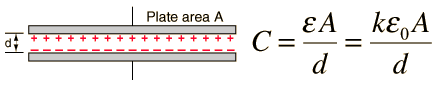Show

The capacitance of flat, parallel metallic plates of area A and separation d is given by the expression above where:= permittivity of space and
k = relative permittivity of the dielectric material between the plates.

k=1 for free space, k>1 for all media, approximately =1 for air.

The Farad, F, is the SI unit for capacitance, and from the definition of capacitance is seen to be equal to a Coulomb/Volt.

Any of the active parameters in the expression below can be calculated by clicking on it. Default values will be provided for any parameters left unspecified, but all parameters can be changed. After editing data, you must click on the desired parameter to calculate; values will not automatically be forced to be consistent.

 Capacitance = k x ε0 x area / separation

For parallel plates of area A = m2 and separation d = m,
with relative permittivity k= , the capacitance is

 C = μF = x10^ F = pF
Index

Capacitor Concepts

 HyperPhysics***** Electricity and Magnetism R Nave
Go Back

# Capacitance of Parallel Plates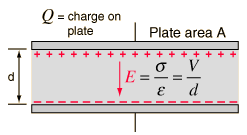The electric field between two large parallel plates is given by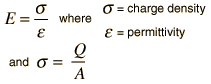Show

The voltage difference between the two plates can be expressed in terms of the work done on a positive test charge q when it moves from the positive to the negative plate.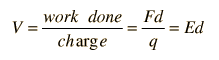It then follows from the definition of capacitance that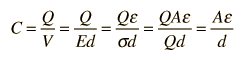Calculation Effect of dielectric What is the dielectric constant?
Index

Capacitor Concepts

 HyperPhysics***** Electricity and Magnetism R Nave
Go Back